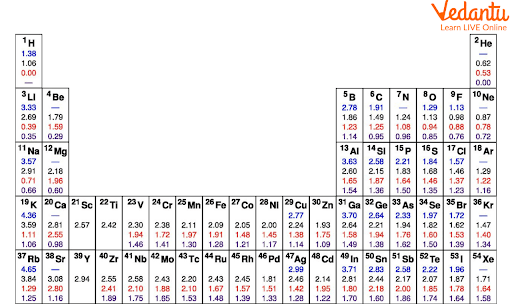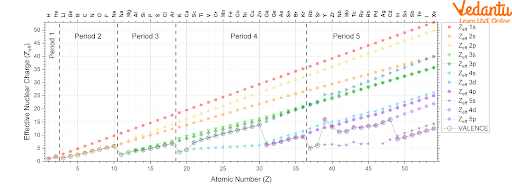Courses
Courses for Kids
Free study material
Offline Centres
More

# Effective Nuclear Charge for JEELast updated date: 25th Nov 2023
Total views: 20.1k
Views today: 0.20k## Introduction

Coulomb’s law is used to predict the attractive or repulsive force between two charged bodies and it is directly proportional to the product of charges and inversely proportional to the square of the distance between them. This law can be used to predict the energy of electrons in hydrogen which has one proton in the nucleus and one electron and in hydrogen like atoms, e.g.,  Helium ion. However, this law cannot be used to predict the energies of electrons in multi-electron atoms and ions.

In a multi-electron atom, the electron in question has to interact with the nucleus and with all other electrons. Thus, each electron in such systems experiences two types of force: (1) attraction to the nucleus and (2) repulsion from other electrons. The presence of multiple electrons results in more repulsive force and this decreases the nuclear attraction to some extent. Thus, the amount of nuclear charge or positive charge experienced by an electron when it is present in a multielectron atom or ion is called effective nuclear charge.

## What is Effective Nuclear Charge?

The energy of an electron in a multi-electron atom depends on both its principal quantum number(shell) and on its azimuthal quantum number(subshell). In a given principal quantum number, the energy of orbitals increases in the order s<p<d<f. But for higher energy levels, there are differences in the energy of orbitals and it will not follow the above order.

For example,  4s < 3d and 6s < 5d ; 4f < 6p.

The different energies of the subshells are due to the mutual repulsion experienced by the electrons.

In a multi-electron atom when the total attractive interactions are more than repulsive interactions, it will be stable. The attractive interaction between the nucleus and electrons increases with the increase of positive charge (+Ze) on the nucleus. This attractive interaction for the outer shell electron will be lower because of a phenomenon called Shielding. The cause of this is the partial screening of the positive charge of the nucleus by the inner shell electron. Due to the shielding of inner-shell electrons, the outer electrons will not have the full experience of the positive charge of the nucleus.

The effective nuclear charge definition is as follows: It is the net positive charge experienced by the outer electrons due to shielding of positive charge by inner shell electrons. The effective nuclear charge is denoted by Zeff .

## Calculation of Effective Nuclear Charge

To calculate, we'll write out an atom's orbitals and divide them into ‘groups’. Each change in shell number creates a new group; the s and p subshells belong to the same group, while the d and f orbitals belong to their own. You write out all of the orbitals in parentheses until you reach the group of the electron-of-interest, as seen below:

(1s), (2s, 2p), (3s, 3p), (3d), (4s, 4p), (4d), (4f), (5s, 5p), and so on.

• Electrons in the Same Group: Every additional electron in the same group as the chosen electron contributes 0.35 to $\sigma$ (do not count the chosen electron).

• If the electron-of-interest is in a d or f subshell, every electron in groups () to the left contributes 1.00 to $\sigma$. This indicates that all electrons in the same shell with a smaller value of l, as well as all electrons in lower shells, shield d and f electrons completely ( n ), due to the poor shielding effect of d electrons.

• All electrons in the next lower shell (n - 1) contribute 0.85 if the electron-of-interest is in an s or p subshell. Furthermore, all electrons in lower shells contribute 1.00 to $\sigma$. This means that s and p electrons are shielded 85% by electrons one shell lower and 100% by all electrons in shells n - 2 and lower.

• 1s electrons $\sigma=0.3$ of 1s electron for every element.

## Slater’s Rules

First, write all the orbitals in the atom until the electron of interest. The orbitals are separated by groups. The new shell number is another group. Also in the same shell number, s and p subshells are in the same group but d and f form their own group. The orbitals must be written based on the increasing energy. The groups are (1s)(2s,2p)(3s,3p)(3d)(4s,4p)(4d)(4f)(5s,5p) etc.

Rules for electrons in the same group as electron-of-interest: Without considering the electron-of-interest, each electron in the same group contributes 0.35 to the shielding constant (S). This means 35% shielding by electrons in the same group with each other.

Rules for electrons to the left of  the group containing  electron of interest:

• If the electron-of-interest is in a d or f subshell, then all electrons in groups to the left of the group having electron-of-interest contribute 1.00 to the shielding constant. This means 100% shielding of d or f subshell electrons by inner shell electrons.

• When the electron-of-interest is in an s or p subshell, the electrons in (n-1) contribute 0.85 to s and the lower shell electrons 1.00 to s.

1s electrons in an atom always have a shielding constant of 0.3.

Consider a Neon atom, it has nuclear charge 10 (Z=10) and electronic configuration 1s22s22p6.

The group will be (1s2) (2s22p6).

If the electron-of-interest is 2p electron. Based on the rules,

then S = 2 ×  0.85 + 7 × 0.35 = 4.15

Zeff  = Z - S = 10 - 4.15 = 4.85

Now, let us calculate Zeff for a 3d-electron in a zinc (Zn, Z= 30) atom.

The groups are (1s2) (2s22p6)(3s23p6)(3d10)

S = 0.35×9+1×18 = 21.15

Zeff  = Z - S = 30 - 21.15 = 8.85

## Values of Effective Nuclear Charge

The effective nuclear charge table shows the value of effective nuclear charge for different elements.Values for Effective Nuclear Charge Table

## Effective Nuclear Charge Trends

Zeff in a specific shell or subshell- The Zeff for electrons in a given shell and subshell increases as the atomic number increases; this tendency is observed both across and down the periodic table.

Understanding trends in valence Zeff is important for valence electrons since the valence Zeff determines atomic/ionic characteristics and chemical reactivity. As the valence shell and/or subshell change as the atomic number increases, the trends in the valence Zeff are not simple.

Down the Group:- As we go down the group of the periodic table, the valence  Zeff increases as the atomic number increases down the group.

Along a period:

Periods 1-3 (s and p only): As we go across periods 1-3, the shell remains constant as Z increases and the subshell changes from s to p. In these periods, there is a gradual increase in valence Zeff. For example, the effective nuclear charge of magnesium is 3.31 at the periphery while the effective nuclear charge of chlorine is 6.12 at the periphery. This indicates that Zeff increases along a period.

In periods 4 and 5 in the d subshell, effective nuclear charge shows an exceptional change in 4d subshell.Trend of the Effective Nuclear Charge with Atomic Number

## Conclusion

A nuclear charge is equal to the electric charge of a nucleus of an atom. The effective nuclear charge is the net attractive positive charge of nuclear protons acting on the electrons in a multi-electron atom or ion. This will be always less than the actual nuclear charge due to the shielding effect. The shielding effect means the decrease of the attractive electrostatic interactions between nuclear protons and valence electrons due to the partially or fully filled inner shells. This effect increases as the number of inner shells of electrons increases. The calculation of effective nuclear charge requires the value of shielding constant which can be determined by Slater’s rules.

## FAQs on Effective Nuclear Charge for JEE

1. What happens to the ionisation energy as we move down a group? Explain.

Ionisation energy is the energy required to remove a valence electron from an atom. It decreases down a group. The shielding effect on the valence electrons is more when an atom has a larger atomic radius. As we move down the group, atomic radii increases. Hence, the shielding effect is more as we move down a group. Here, the increasing atomic number results in more inner shell electrons which block the valence electrons from feeling the pull towards the nucleus. Hence, valence electrons can be easily removed and this causes a decrease in the ionisation energy.

2. Why does effective nuclear charge increase from left to right in a periodic table?

Generally, the effective nuclear charge increases from left to right across the periodic table because there is an increase in atomic charge and a constant shielding effect. Due to the constant shielding effect,  the valence electrons are pulled more tightly to the nucleus. This creates a smaller atomic radius, higher ionisation energy, and higher net positive charge on the atom as we move across the periodic table.

This is more pronounced in periods 1-3 and there is a gradual increase in valence electron effective nuclear charge. For periods 4 and 5 electron configuration is going from  (n)s subshell to (n−1)d subshell, there is a relatively large increase in valence Zeff, and from (n-1) d to np sub-shell, there is a relatively large decrease in valence Zeff.

3. In the three isoelectronic species of fluorine anion (F-), neutral neon atom (Ne), and sodium cation (Na+), what is the Zeff experienced by the valence electrons?

Each species contains ten electrons, with two core electrons (10 total electrons - eight valence), but the effective nuclear charge differs due to atomic number differences (Z). Slater's rules can be used to find the approximate Zeff. We would calculate the same sigma value for all of these species:

Using the formula, the screening constant turns out to be  (1s)(2s,2p), =2(0.85)+7(0.35)=1.7+2.45=4.15

$\sigma =4.15$

The anion of fluorine: Zeff=9-4.15=4.85

Zeff=10-4.15=5.85 (for the neon atom)

Zeff=11-4.15=6.85( for sodium cation)

As a result, the sodium cation has the highest nuclear charge.

4. What do you mean by calculating effective nuclear charge at the periphery?

Periphery refers to the end, which implies that the effective nuclear charge is calculated at the border including all the electrons in the element. The problem is solved by Slater's rule applying the formula for Zeff.  For example, when an additional electron is introduced to a nitrogen atom at the periphery, 7  electrons shield at the periphery in the second orbit (2s22p4) and two electrons in the first orbit (1s2). Hence further using the normal rules and formula $Z_{eff}=Z-\sigma$  an effective nuclear charge can be calculated.

5. What is the difference between nuclear charge and effective nuclear charge?

• Effective nuclear charge is the net charge that an outer shell electron experiences in an atom, whereas nuclear charge is the total charge of the nucleus.

• While effective nuclear charge is determined taking into account the influence of inner orbital electrons and the nuclear charge, nuclear charge is independent of the charge of electrons in an atom.

• Effective nuclear charge does not solely depend on the number of protons in the nucleus, whereas nuclear charge solely depends on the number of protons present in the nucleus.